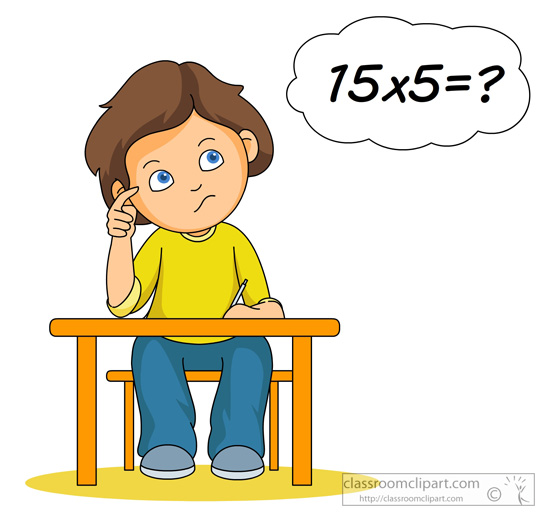# Long multiplication – which method works best?In the latest Building Bridges article for NCETM (link below), I discuss the merits of some well-known methods for long multiplication:

NCETM – Long multiplication by lattice method

Let me know what you think and also check out my previous post on times tables as these skills are the cornerstone of long multiplication.

If you don’t have an NCETM login, here’s the full version of the article:

## Building Bridges – Issue 129

In the last issue, we discussed strategies for developing pupils’ fluency with their times tables. Once these key facts are secure, how can we improve our pupils’ fluency with procedures that use them, in particular “long multiplication”? We discussed last year (in Issue 119), the grid method, and advocated it as a powerful and long-lasting model that helps pupils extend their conceptual understanding from area and the multiplication of numbers to the multiplication and factorization of algebraic expressions. A challenge to the grid method is that it’s not very efficient for multiplying two 3-digit or larger numbers, and that it requires pupils to be confident with place value: it’s a good stepping stone from concrete to abstract multiplication, but it’s often not the best method when actually you want to calculate the product of two numbers. The traditional “long multiplication” algorithm is more efficient than the grid method, but it too expects confident understanding of place value: in a KS3 class with pupils with low prior attainment, that may be an unrealistic expectation, at least when they first join the school.

An algorithm which takes away the stress of being forced to consider place value (which will result in errors if zeros are forgotten or misunderstood and hence misplaced), is to use what’s often called the Lattice Method. We must point out here, for historical accuracy, that this is sometimes incorrectly referred to as Napier’s Method. Napier did devise a clever system of multiplication using blocks (known as Napier’s Bones) built on the Lattice Method; this is a story well worth investigating further. A strong advantage of the Lattice Method is that it requires, and hence reinforces, understanding of and fluency with times tables up to 9, and most of the place value thinking is ‘below the surface’. Pupils, especially those with low prior attainment, therefore often find it an easier algorithm to implement correctly than the grid method or classic “long multiplication”; this builds their confidence, which is hugely important. The lattice method helps pupils get the right answer without getting bogged down by other concepts which may not be securely understood, or which may be understood but in practice can still produce errors.

The beauty of the algorithm is in its simplicity: let’s take an example and calculate 23 × 385 = 8855. We multiply the digits pairwise, and record the answer to each separate multiplication using tens and units above and below the diagonal lines:It can also be used effectively to multiply two decimals such as 1.58 × 1.6:Of course, the decimal point can be inserted after calculating first the integer product 158 × 16, and then considering the scale factors between the original factors, the integers, the integer product, and hence the original product. There are short videos on YouTube showing lattice multiplication and decimal lattice multiplication.

The caveat to the lattice model is no doubt obvious, but let us state it nonetheless: the lattice method is a good algorithm, but it’s not a good model for multiplication – in particular, it doesn’t generalise naturally from the concrete to the abstract. Therefore, once pupils are confidently calculating products using a lattice, they must then also calculate them using a grid and a “long multiplication”, so that they develop understanding of and fluency with these more powerful models.

Also, this is a good context for developing pupils’ reasoning: they can consider a rich question such as “what’s the same and what’s different?” about the three methods as they are written out on the page – comparing the lattice above with

 300 80 5 × 6000 1600 100 20 900 240 15 3 6900 1840 115 8855

and

 3 8 5 × 2 3 1 1 25 15 7 17 10 0 8 8 5 5

In particular, they should look at the “partway” calculations – 6900, 1840 and 115 in the grid method and 1155 and 7700 in the long multiplication. Do these also occur, perhaps hidden, in the lattice? If not, is just a miracle that the answers are all the same (we hope!)?